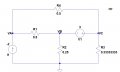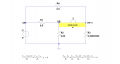# Am I doing this nodal analysis correctly? (SuperNode)

#### Nuno Fernandes

Joined Feb 24, 2016
9
Hello!
I'm having trouble solving a problem with the circuit below. I am supposed to find Va, Vb and Vc through nodal analysis.
I got to these three equations (in the picture, where it says that R3 = 0.333..., it is supposed to be R3 = 1/3):
(Va-Vc)/0.5 + (Va-Vb)/0.5 +2 = 0;
(Vb-Va)/0.5 + Vb/0.25 + (Vc-Va)/0.5 + Vc/(1/3) = 0;
Vc-Va = 3;

I can't get to the correct values I got on the simulation. Is it a problem with the KCL equations? Thank you.#### StayatHomeElectronics

Joined Sep 25, 2008
1,073
The last equation is not correct. The voltage source is between which two voltages?

#### WBahn

Joined Mar 31, 2012
26,903
Hello!
I'm having trouble solving a problem with the circuit below. I am supposed to find Va, Vb and Vc through nodal analysis.
I got to these three equations (in the picture, where it says that R3 = 0.333..., it is supposed to be R3 = 1/3):
(Va-Vc)/0.5 + (Va-Vb)/0.5 +2 = 0;
(Vb-Va)/0.5 + Vb/0.25 + (Vc-Va)/0.5 + Vc/(1/3) = 0;
Vc-Va = 3;
One habit you want to get into is checking your own set-up equations before you start solving anything. If the set-up isn't correct, then the answers can't be. Remember, the physics and electrical engineering is all in the set-up -- everything else is just math.

You also need to track your units properly -- most mistakes you make will mess up the units allowing you to catch them, but only if the units are being properly used so that they are there to get messed up.

(Va-Vc)/(0.5 Ω) + (Va-Vb)/(0.5 Ω) + 2 A = 0;
(Vb-Va)/(0.5 Ω) + Vb/(0.25 Ω) + (Vc-Va)/(0.5 Ω) + Vc/(1 Ω/3) = 0;
Vc - Va = 3 V;

Go through each node equation (your first two equations) and picture the specific current that each term represents, including which way it is flowing. Ask yourself if those terms properly account for all the current at that node/supernode.

Go through each constraint equation (your third equation) and ask if it represents the correct relationship between the given nodes.

I can't stress enough the importance of checking your set-up equations before proceeding. The whole game is contained in those equations -- those equations ARE both the problem AND the solution. Never assume that you did them correctly. Always check them after you have gotten them written down in order to verify that you didn't mess up -- because you WILL mess up on a regular basis, we all do.

•Nuno Fernandes

#### RBR1317

Joined Nov 13, 2010
701
This is a good opportunity to explore an alternate method of solution using a super-node. Whenever two node voltages are separated by a known offset, those two nodes can be combined into a super-node. Only one node equation needs to be written for a super-node versus the two equations for the individual nodes. The caveat is that the individual offset node voltages must be used in the node equation as appropriate. To ensure that this is done correctly, mark the offset voltages directly on the diagram.

For this problem I have created the VB super-node, and replaced VC with the VB offset (although it could have been the other way around.) That yields the VA & VB node equations.#### Nuno Fernandes

Joined Feb 24, 2016
9
This is a good opportunity to explore an alternate method of solution using a super-node. Whenever two node voltages are separated by a known offset, those two nodes can be combined into a super-node. Only one node equation needs to be written for a super-node versus the two equations for the individual nodes. The caveat is that the individual offset node voltages must be used in the node equation as appropriate. To ensure that this is done correctly, mark the offset voltages directly on the diagram.

For this problem I have created the VB super-node, and replaced VC with the VB offset (although it could have been the other way around.) That yields the VA & VB node equations.
View attachment 102427
I'm sorry everyone. It was late and I was really tired and didn't even noticed I had the third equation wrong. Noob mistakeThank you for your reply.

#### anhnha

Joined Apr 19, 2012
901
I'm sorry everyone. It was late and I was really tired and didn't even noticed I had the third equation wrong. Noob mistakeThank you for your reply.
And solve for the equations you should get Va = -(4/7), Vb = -(11/7), Vc = 10/7.

#### WBahn

Joined Mar 31, 2012
26,903
I'm sorry everyone. It was late and I was really tired and didn't even noticed I had the third equation wrong. Noob mistakeThank you for your reply.
Which underscores the value and importance of getting in the habit of always (not sometimes, but always) verifying your set-up equations before proceeding. It actually wasn't a noob mistake -- there will always be times when you are tired, or hungry, or hurried, or stressed, or just plain ole a little sloppy. That will be true throughout your career. So you want to set up best practices that, once they become engrained habit, will let you automatically catch most of your mistakes regardless of why they got made.